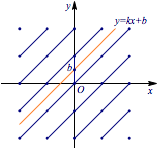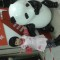每日一题代数与几何结合解决整点问题

①存在这样的直线，既不与坐标轴平行又不经过任何整点；

②如果 $k$ 与 $b$ 都是无理数，则直线 $y=kx+b$ 不经过任何整点；

③直线 $l$ 经过无穷多个整点，当且仅当 $l$ 经过两个不同的整点；

④直线 $y=kx+b$ 经过无穷多个整点的充分必要条件是：$k$ 与 $b$ 都是有理数；

⑤存在恰经过一个整点的直线．

考虑①，画出原点附近的整点，如图的直线既不与坐标轴平行，又不经过任何整点，比如直线$y=x+\dfrac 12$，这样的直线有无穷多条，①成立；$k$ $b$ 整点个数 有理数 有理数 0个或无穷多个 有理数 无理数 0个 无理数 有理数 0个或1个 无理数 无理数 0个或1个关于weilew

2 Responses to 每日一题代数与几何结合解决整点问题

1.Seeker说：

老师这道题标答对3的解释是 若整点(m.n)(s.t)在直线上则对任意整数k都有整点(m+ks.n+kt)在直线上。这样是对您对称点解释的另一种形式吗？或者另有其原因，亦或是他解释有误？

2.朱飞说：

希望多点曲线系解决圆曲的贴